SYLLABUS  Previous: 5.1.1 Term structure models  Up: 5.1 Discound bonds  Next: 5.2 Credit derivatives

5.1.2 Parameters illustrated with VMARKET experiments

[ SLIDE parameters - discounting - price of risk - drifts || VIDEO modem - LAN - DSL ]

Since the terminal value of the discount function at the maturity is simply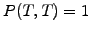, the parameters characterize either the forecast of the spot rate or the numerical method that will be examined later in sect.5.3.1. The financial parameters that are relevant in the applet are:

• the lifetime or maturity date (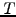or RunTime) of a bond in years, e.g. 10 for a bond reaching its maturity in a decade,
• the maximum volatility (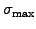or Volatility) of a bond estimated from historical values, e.g. 0.02 for a two percent volatility peak that will be reached after one third of the bond lifetime (5.1.1#fig.1),
• the market price of risk (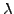or MktPriceRsk) measuring the reward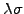expected by the investors for taking an investment risk, e.g. -0.25 in a risk averse market with little appetite for risk. In the applet, the effect is further modulated by a cosine function reproducing (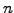or UserDouble) economic cycles during the lifetime of the bond,
• the mean reversion target rate (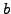or MeanRevTarg) is the value towards which the spot rate returns to after a long time, e.g. 0.05 for a market with a 5% average rate,
• the mean reversion velocity (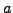or MeanRevVelo) measures the speed of the process, e.g. 0.5 [1/year] for a mean reversion taking about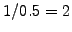years.
• the spot rate (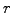or SpotRate) used to plot the term structure of the interest rates.
To visualize the evolution of a bond and the corresponding yield in a very simple case, the VMARKET applet below shows what happens in the absence of drifts (right hand side of 5.1.1#eq.5 equals zero) and without volatility.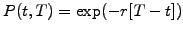as expected for a risk free investment (1.3#eq.6). The bond yield is equal to the spot rate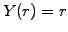and the term structure of the interest rates is constant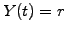.

 Virtual market experiments: trivial bond Vary the length of the simulation domain MeshLength and, by clicking in the plot area, verify that bond yield is indeed equal to the spot rate. Modify the time to the maturity RunTime and verify that you have properly understand all three graphs that are plotted.

The second applet below illustrates the effect of a large volatility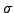in the spot rate and accounts for the extra return investors expect from the market through the so-called market price of risk.

Although this is not immediately apparent in the simulation, the main effect of the volatility is to reduce the curvature of the discount function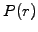by smearing out irregularities in the yield curves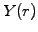,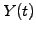: if the forecast rate changes rapidly, the yield curves do not follow immediately everywhere. The reward payed to the investor who accepts the risk associated with fluctuations in the spot rate is clearly visible, with an effective yield that increases with time for a positive value of the market price of risk.

 Virtual market experiments: volatility and the market price of risk Vary the amount of volatility in the spot rate and observe how the effect evolves with time. Remember that 4% volatility is huge for credit markets! Change the value and the sign of the market price of riskassociated in the applet with the MktPriceRsk parameter. Set UserDouble=2 to model two cycles in an economy. Try to identify when the forecasted rates are high; how is the corresponding yield?

The applet above illustrates the effect of evolving drifts in the forecast rates, here modeled with two economic cycles during the lifetime of the bond: recession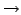cut rateover-heated economyrise rate... or rather the opposite when the time runs backward in the applet.

The third applet below finally illustrates the effect of a mean reversion, which accounts for the tendency of the forecasted rates to fall back to a long term average value.

 Virtual market experiments: mean reversion Change the MeanRevVelo parameterto modify the typical time scale for interest rates to revert back to the target level MeanRevTarg. Use all the forecasting parameters to approximate the price of a bond during 10 years reproducing the evolution of interest rates in your country.

Experimenting with the applet enables you to develop an intuitive understanding for the fundamental processes that characterize the credit market. The experiments also prepare you also for the inverse problem, where the term structure of the interest rates is known from the market (e.g. 2.2.2#fig.1) and the drift / volatility parameters are matched in order to extrapolate into the future (exercise 5.01).

SYLLABUS  Previous: 5.1.1 Term structure models  Up: 5.1 Discound bonds  Next: 5.2 Credit derivatives